Chapter 8: chemical kinetics – relaxation to the equilibrium

Reaction of order 1

Considering an equilibrium between two species A and B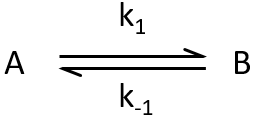We can study the return to the equilibrium (or relaxation) after a sudden modification of the conditions such as a jump of temperature or of pressure, or the modification of an electric field. The kinetics of the relaxation towards the equilibrium adapts to the new conditions.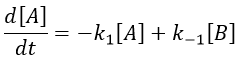The concentrations can be noted as perturbations Δ[I] of the concentrations at equilibrium [I]e.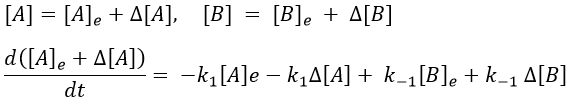[A]e and [B]e don’t vary with the time. And at the equilibrium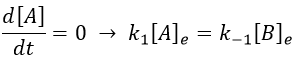As the stoichiometric constants of the species are equal, we can write that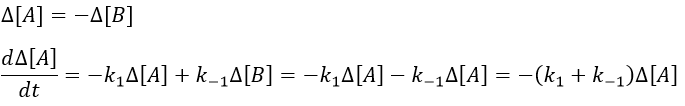This equation can be solved.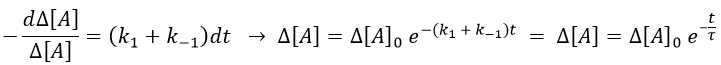The relaxation is thus a decreasing exponential. The sign of the difference of concentration doesn’t matter: the equilibrium can be perturbed towards A or towards B, the relaxation will act the same way.

Reaction of order 2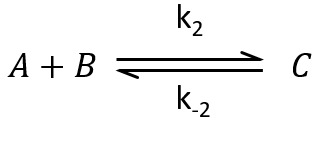The methodology to determine the relaxation of this equilibrium is the same as for an order 1 reaction: we determine the speed of the reaction and insert Δ[X] in this expression. We use the properties that the concentrations are constant at the equilibrium and that only a small perturbation is applied to the system. This last assumption leads to a simplification of perturbations of order 2 or more (such as Δ[A]2 or ∆[A]∆[B]).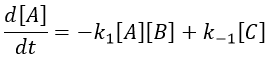At the equilibrium, we have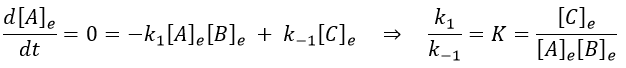Inserting the perturbations in the expression of the speed, we obtain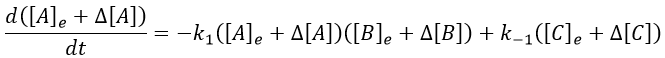In the development of [A][B], we can ignore the ∆[A]∆[B] term because it is very small in comparison to the other terms. Knowing the stoichiometric coefficients, we know the relation between the perturbations in concentration.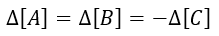It leads to the following expression.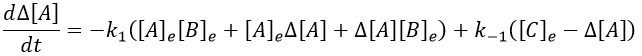We can simplify the expression with the relation between the concentrations at the equilibrium.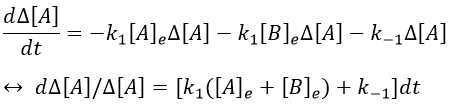As a result,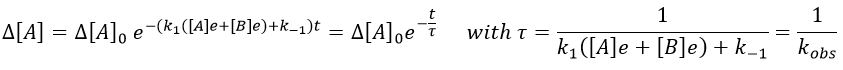We can thus find the constants of speed of the reaction from the relaxation of the system that follows a perturbation. To do so, we repeat the experiment with several compositions of reactants. On the graph 1/τ vs [A]+[B], k1 and k-1 can be determined.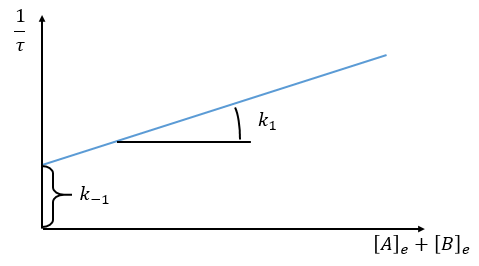Optical measurement of the distance to the equilibrium

The relaxation is thus given by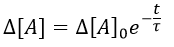If we want to follow the process optically, we can measure the absorbance α of the solution at a given wavelength.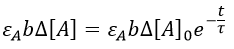It can be difficult to find a wavelength where only one species absorbs and not the others so we simply measure the absorbance of the solution at one wavelength even if it is a combination of the absorbance of several species.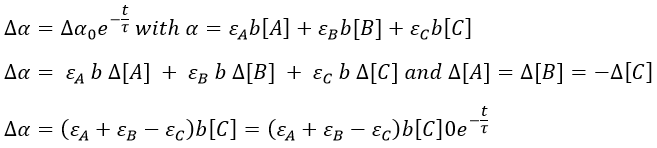The precision will be the best if εABC is large. We can check the intensity of the signal while at the equilibrium to find a wavelength for which the signal is strong.

Relaxation followed by conductivity

We can use this method of measurement if there is a perturbation of the ionic equilibrium of the solution. For instance in an acid-base reaction,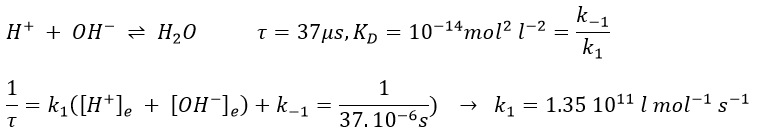If the reaction has been driven by diffusion, we hould have found a relaxation period around 10-9-10-10 l mol-1s-1. The relaxation here is faster because the proton doesn’t properly diffuse. Instead, the proton-hopping phenomenon applies in aqueous solutions: each oxygen atom simultaneously passes and receives a single hydrogen atom that diffuses through the hydrogen bond network of water molecules.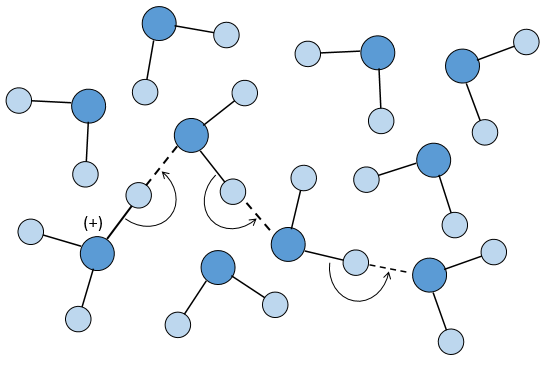Application: study of relaxation of the complex proflavin-DNA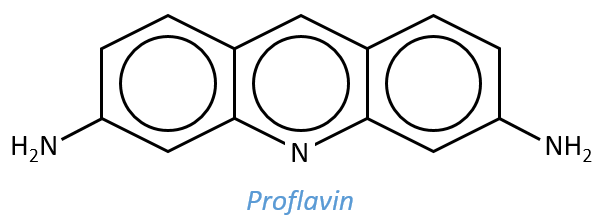The proflavin is a mutagen agent that can insert itself in the double helix of the DNA. It is an amphoteric species the heteroaromatic N of which is protonated at pH=7. The mechanism of interaction between the proflavin and the DNA is complex and not only driven by chemical processes.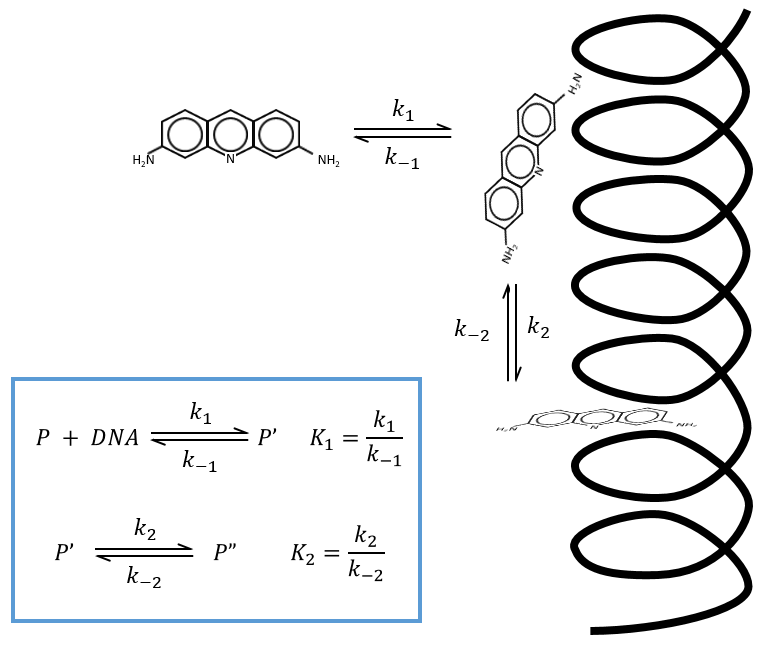The supposed mechanism is that the proflavin diffuses near the DNA double helix in a first step. Once it is there, there is an interaction between the NH+ and the phosphates of the helix. In a last step the proflavin inserts itself in the DNA helix that had to open to let the mutagen agent enter. It may cause errors during the replication of the DNA by enzymes. We will note P the proflavin far from the helix of DNA, P’ along the helix and P” inside the helix.

If we impose a jump of temperature (~1micros) to the system when it is in equilibrium, we can measure the relaxation by absorbance. We observe that there are two relaxation times of different scales: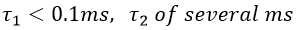We can assume that there are two equilibriums involved in the process.

If the two reactions had been independent, then we would be able to use the expressions that we have find earlier in this chapter. However P’ is involved in the two equilibrium. Yet, the relaxation times are of different scales. If the first step is very fast in comparison to the second step, we can consider that the equilibrium K1 is reached. A quantity x of P and of DNA has been “consumed” in the first equilibrium (in the case of the DNA, consumed means that there is no place to bind with it on a given portion of the helix, one branch of DNA is capable to accept several proflavin molecules in its helix). A quantity z of P” is formed by the second reaction and a quantity y=z-x of P’ is consumed in total (y can be positive or negative).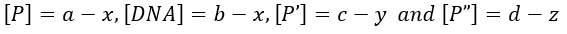As the first equilibrium is always reached over the relaxation period τ2 of the second reaction,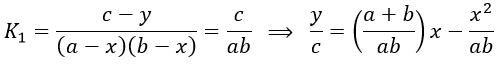The x2 term can be neglected (small perturbation).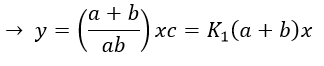x=z-y so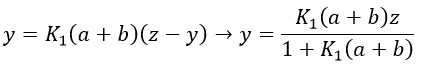P” is involved only in the last step of the reaction which is slow.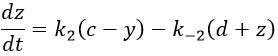The value of y can be inserted in the equation to find that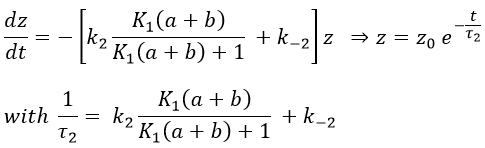If the steps had been independent, we would have expected 1/τ2=k2+k-2 but there is a correction on the constant of speed k2 that involves P’ (which takes part in both reactions).

If K1(a+b)>>1, then the reactions can be considered as independent (1/τ2=k2+k-2)

If K1(a+b)<<1, then the relaxation time of the process is smaller than for independent reactions (τ2,coupled< τ2,indep)

If K1(a+b)<<<1, then the relaxation time is much larger than for the independent process (τ2»1/k-2).

We can play on the concentrations a and b to determine the constants of speed.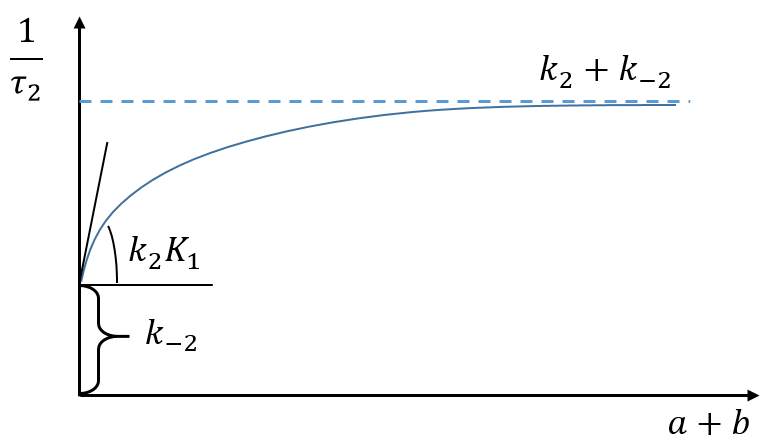Experimentally, values have been determined for a pH=6.9, a temperature of 10°C and a concentration of Na+ of 0.2M:

k1=107 M-1s-1, a value corresponding to a diffusion driven process with a heavy molecule

k-1=3.5 103 s-1. This step is much slower than the diffusion because there is an electrostatic attraction between the helix of DNA and the proflavin. The dissociation is made without modification of the conformation of the DNA

k2=1.5 103 s-1 and k-2=1.1 103 s-1. We have a confirmation that the second step of the reaction is slower than the first step. It is longer to get inside the helix than to approach it. Note that it is slightly faster to move inside the helix than outside. The helix is disorganised and it is favourable from the entropic point of view.

Chain reactions

This section is useful for polymeric and catalysed reactions (for instance). In these reactions we discern three main steps: initiation, propagation and termination.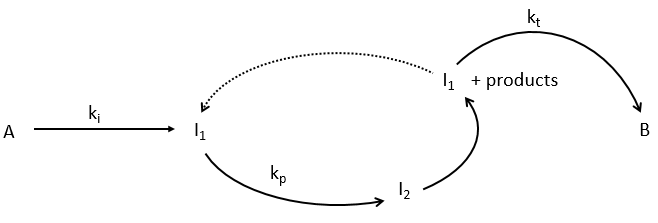We will begin this section with the example of the formation of ortho-hydrogen from para-hydrogen (antiparallel nuclear spin and parallel nuclear spin). For this reaction (at 100°C), we find an order of 3/2.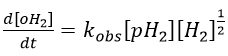with H2 the total concentration of hydrogen (ortho and para). As soon as we see a concentration with a power of (2n+1)/2, we can assume that the mechanism is not elementary. The mechanism is thus more complex than a simple equilibrium. An additional species is required to proceed to the inversion of spin: a catalyst noted M.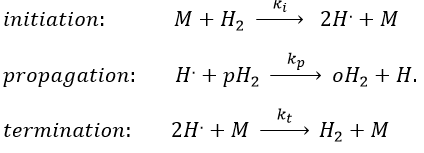The propagation step can be repeated indefinitely as long as there is some pH2 in the system. Eventually, two radicals react together. The evolution of the concentration in radicals is given by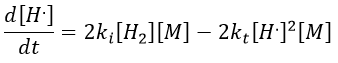If we consider that [H.] is stationary, then we can find its value.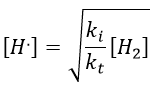We can insert this value in the expression of the concentration of oH2.It corresponds to the experimental observation.

Example of HBr condensation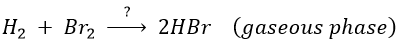This reaction is not an elementary reaction and is in fact the result of a chain reaction. It is indeed hard to imagine that the two diatomic molecules that have not the same length of liaison, are perfectly aligned and vibrate in phase. The speed of the reaction can be determined experimentally: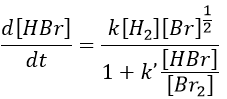One can see that the product of the reaction is in the denominator of the equation, meaning that the speed of the reaction decreases with the concentration of the product. The explanation is that here should be an inhibition step in the process that consumes the product.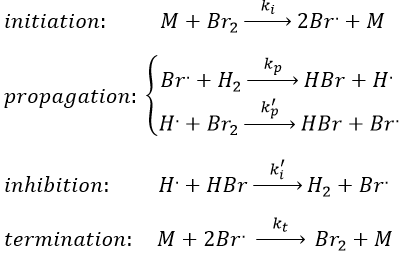Note that the inhibition and termination steps are in fact the opposite reactions of the initiation and of the first reaction of propagation (kt=k-i and k’i=k-p). M is a catalyst on the surface of which the reaction occurs. Looking at the balance of the product, we have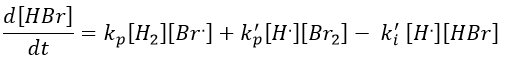We will link this equation with the experimental expression of the speed by assuming the stationarity of the concentration of radicals H. and Br..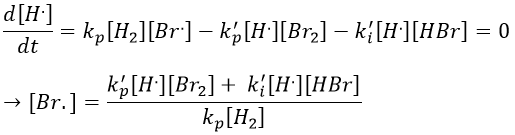This expression still involves the concentration of a radical that is not measurable. We can insert this expression of [Br.] in the balance of the product.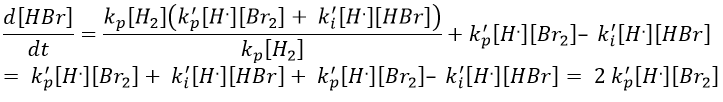We do the same with the second radical.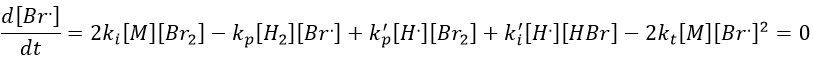We can determine the concentration of Br. if we sum the variations of concentration of the radicals (still equal to zero):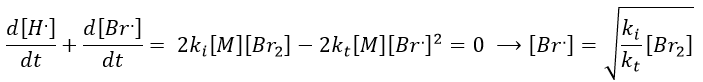We can insert this expression in d[H.]/dt to obtain a measurable expression.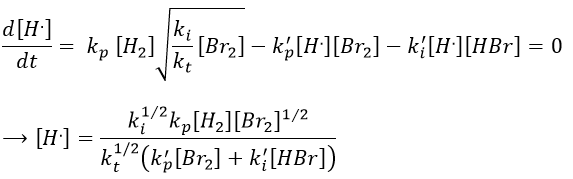We can now express the concentrations of the radicals in the balance of the product HBr.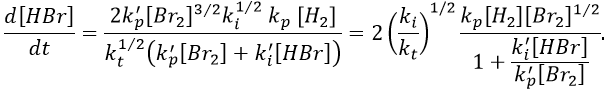We can compare this expression to the experimental observation and conclude that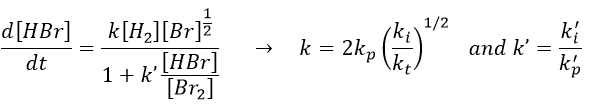k’»0.1 is almost independent of the temperature (both reactions are exothermic and are of small energy). Note that some other reactions were possible and were not written. There is a second reaction of initiation and of inhibition but the energies of dissociation of H2 and HBr are way higher than the one of Br2.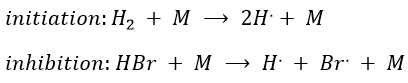Some reactions of termination are also omitted for a different reason: the H. radical is extremely reactive and is active in the propagation step of the reaction. The concentration of this radical is thus very small.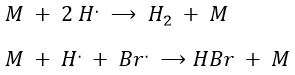Note that those two reactions are the reverse reactions of the previous ones. Finally, there is also another method to initiate the reaction: instead of using a catalyst, we can use a laser (hν) but the termination step still requires M. As a result, the equations change and the kinetics are different.

This scheme of reaction is also valid for Cl2 and F2. In the case of I2, we observe an order 2 reaction kinetics. We have to heat up the system at 800K to obtain 95% of chain reaction.

Case of the stratospheric ozone

In the stratosphere at 20-40km of altitude, there is an equilibrium between the concentrations of O2 and O3. The rays coming from the outer space influence this equilibrium.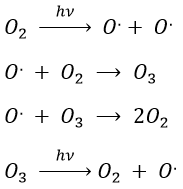The ozone is important at this altitude because it filters the damaging UV rays from the sun. Before 1950, chlorofluorocarbons (CFC, for instance CFCl3, CF2Cl2) were commonly used as propellers in sprays and as coolers in fridges. No particular attention was done for their destruction and the CFC made a slow ascension towards the stratosphere. A hole in the ozone layer started to deepen at the poles (there because the hot ozone diffuses towards the cold areas). A problem is that the time it takes CFC’s to reach the stratosphere can be counted in years and when the phenomenon was detected (1971) a huge quantity of CFC’s were still on their way up.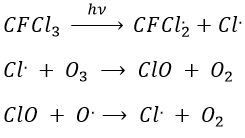As a result, one portion of the ozone was consummed to form ClO and the equilibrium between O2 and O3 was displaced.